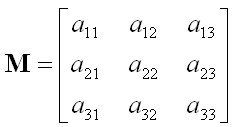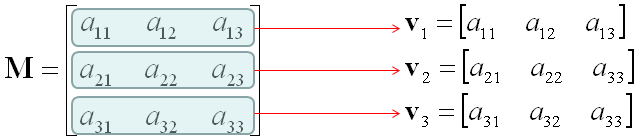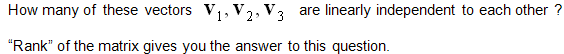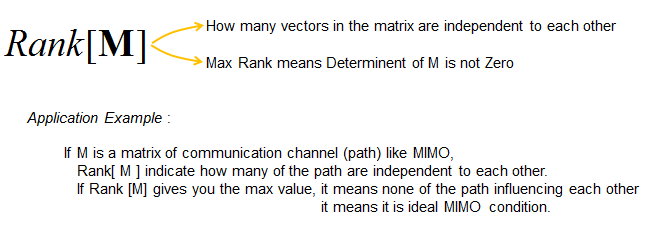Engineering Math - Quick Reference                                 Home : www.sharetechnote.com Matrix- Rank   Rank is an indicator that shows how many of the vectors comprising a matrix are linearly independent to each other. For example, let's suppose we have a matrix as follows.Let's take each row of the matrix and construct vectors as follows. (These vectors are called 'row vector')Now the question is how many of these vectors are linearly independent to each other ? The answer to this question is the Rank of the matrix.(Refer to "Linear Independent" definition)   Probably this definition itself would sound too dry to you. Just remember followings and try to apply when you need.For more intuitive explanation, I want to introduce a good video linked here.